Next: Competitive Hebbian Learning Up: Soft Competitive Learning without Previous: Soft Competitive Learning without

# Neural Gas

The neural gas algorithm (Martinetz and Schulten, 1991) sorts for each input signal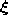the units of the network according to the distance of their reference vectors to. Based on this ``rank order'' a certain number of units is adapted. Both the number of adapted units and the adaptation strength are decreased according to a fixed schedule. The complete neural gas algorithm is the following:
1.
Initialize the setto contain N unitswith reference vectors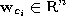chosen randomly according to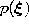.

Initialize the time parameter t: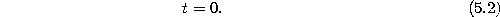2.
Generate at random an input signalaccording to.
3.
Order all elements ofaccording to their distance to, i.e., find the sequence of indicessuch thatis the reference vector closest to,is the reference vector second-closest toandis the reference vector such that k vectorsexist with. Following Martinetz et al. (1993) we denote withthe number k associated with.
4.
Adapt the reference vectors according towith the following time-dependencies:5.
Increase the time parameter t:6.
If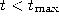continue with step 2

For the time-dependent parameters suitable initial valuesand final values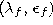have to be chosen. Figure 5.1 shows some stages of a simulation for a simple ring-shaped data distribution. Figure 5.2 displays the final results after 40000 adaptation steps for three other distribution. Following Martinetz et al. (1993) we used the following parameters:.Figure 5.1:   Neural gas simulation sequence for a ring-shaped uniform probability distribution. a) Initial state. b-f) Intermediate states. g) Final state. h) Voronoi tessellation corresponding to the final state. Initially strong neighborhood interaction leads to a clustering of the reference vectors which then relaxes until at the end a rather even distribution of reference vectors is found.Figure:   Neural gas simulation results after 40000 input signals for three different probability distributions (described in the caption of figure 4.4).Next: Competitive Hebbian Learning Up: Soft Competitive Learning without Previous: Soft Competitive Learning without

Bernd Fritzke
Sat Apr 5 18:17:58 MET DST 1997